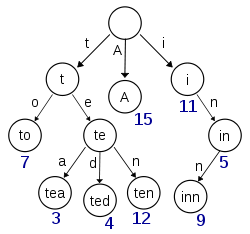• ## Trie

2020-03-15 16:26:27
1.Trie的概念： Trie又称字典树，前缀树（事实上前缀树这个名字就很好的解释了Trie的储存方式） 来一张图理解一下Trie的储存方式：(图片来自百度百科) 通过这张图，我们可以看出Trie的特点： Trie没有根节点，也...
1.Trie的概念：
Trie又称字典树，前缀树（事实上前缀树这个名字就很好的解释了Trie的储存方式）
来一张图理解一下Trie的储存方式：(图片来自百度百科)通过这张图，我们可以看出Trie的特点：

Trie没有根节点，也就是无根树。
除根节点外，每一个节点的会储存一个字母或单词！
从根节点到每个单词节点的路径上的所有字母连接而成的字符串就是该节点对应的字符串
每个非叶子结点一般都会被多次使用，以节省遍历时的时间效率、
另外，每个节点下面的数字是他们的编号，这个具体在下面再展开

所以我们可以看出，这是典型的空间换时间
2.trie的操作

常用的操作一般有：
查找（find），插入（insert），删除（isFreeNode）
这里删除实在是太少见了，所以不展开讨论。

那些非根节点下面的数字是什么意思？
事实上，在代码中，我们一般把根节点编号为0，然后把其余节点从1开始编号，然后存在一个数组中（数组的用处是储存每个节点的所有子节点，以便于直接使用下标来存取）
再具体一点，在我的代码中，用trie[u]（结构体）中son[v]的来保存u的那个编号为v的子节点，当然，如果trie[u].son[v]==0，也就代表这个节点不存在，即u没有这个编号为v的子节点
填完了坑，我们接下来讲（查找和插入）

前言：一般一道关于Trie的题目，不会只让你去单纯的构造一棵
Trie树（字典树），一定会有一些附加信息，我们一般根据题目
意思来定义一个变量，这里我们举一道模板题来加深理解

题目原题
题目大意：给你n个名字（name），然后叫你点m的名字，问你
对于你每点一次名字，这个名字有没有重复点过（输出“REPEAT”）
反之输出”OK“，还有如果这个名字不正确，也就是它不是n个名字
中的名字（输出“WRONG”）
插入：
这道题是叫我们求得附加信息是有没有出现过，我们用have来
表示。
我们先讲讲我们要用的变量及数组
//cnt表示这个名字是出现了几次
//son数组表示这个节点的所有情况（因为全是小写字母，所以有26个）
//表示这个名字是否是n个名字中的名字
struct node
{
int cnt,son;
bool have;
node() //初始化
{
cnt=0;
memset(son,0,sizeof(son));
have=0;
}
} trie;
//因为我们是以一个名字的每一个字母为一层，所以是n*每个名字最长的长度（50位）
然后我们为了保险，再定大点-_-

code（具体解释请看程序）：
void insert(char s[])
//这里我没有用指针这种不友好的东西，当然你也可以用*s
{
int u=0,v,len=strlen(s);
for(int i=0;i<len;i++)
{
v=s[i]-'a'; //取得它的编号
if(!trie[u].son[v]) trie[u].son[v]=++sum;
//表示这个点没有赋值过
u=trie[u].son[v];
//继续遍历下一个
}
trie[u].have=true;  //将这个单词标识为出现过
}

查找：
code
//与上边同理
//return 1;表示这个名字合理并且第一次出现
//return 2;表示这个名字合理并且重复出现
//return 3;表示这个名字不合理（不在这n的名字中）
int find(char s[])
{
int u,v,len=strlen(s);
for(int i=0;i<len;i++)
{
v=s[i]-'a';
if(!trie[u].son[v]) return 3;
//如果这个单词中字母没有存储过，则表示没有这个单词
u=trie[u].son[v];
}
if(!trie[u].have) return 3;
//判断这个名字是否在n个名字中
if(!trie[u].cnt) //判断这个名字是否是第一次出现
{
trie[u].cnt++;
return 1;
}
return 2;
}

完整code
#include<cstdio>
#include<iostream>
#include<string>
#include<cstring>
using namespace std;
struct node
{
int cnt,son;
bool have;
node()
{
cnt=0;
memset(son,0,sizeof(son));
have=0;
}
} trie;
int sum=0;
void insert(char s[])
{
int u=0,v,len=strlen(s);
for(int i=0;i<len;i++)
{
v=s[i]-'a';
if(!trie[u].son[v]) trie[u].son[v]=++sum;
u=trie[u].son[v];
}
trie[u].have=true;
}
int find(char s[])
{
int u,v,len=strlen(s);
for(int i=0;i<len;i++)
{
v=s[i]-'a';
if(!trie[u].son[v]) return 3;
u=trie[u].son[v];
}
if(!trie[u].have) return 3;
if(!trie[u].cnt)
{
trie[u].cnt++;
return 1;
}
return 2;
}
int main()
{
int n,m;
cin>>n;
for(int i=1;i<=n;i++)
{
char name;
cin>>name;
insert(name);
}
cin>>m;
for(int i=1;i<=m;i++)
{
char name;
cin>>name;
int t=find(name);
if(t==1) cout<<"OK"<<endl;
else if(t==2) cout<<"REPEAT"<<endl;
else cout<<"WRONG"<<endl;
}
return 0;
}




展开全文• ## trie

2019-03-23 16:35:06
trie，又称前缀树或字典树。它利用字符串的公共前缀来节约存储空间。 性质 （1）根节点不包含字符，除根节点外的每个节点只包含一个字符。 （2）从根节点到某一个节点，路径上经过的字符连接起来，为该节点对应的...
trie，又称前缀树或字典树。它利用字符串的公共前缀来节约存储空间。
性质
（1）根节点不包含字符，除根节点外的每个节点只包含一个字符。
（2）从根节点到某一个节点，路径上经过的字符连接起来，为该节点对应的字符串。
（3）每个节点的所有子节点包含的字符串不相同。
class Trie(object):

def __init__(self):
"""
"""
self.root = {}
self.word_end = -1

def insert(self, word):
"""
Inserts a word into the trie.
:type word: str
:rtype: None
"""
curNode = self.root
for c in word:
curNode = curNode.setdefault(c,{})
curNode[self.word_end] = True

def search(self, word):
"""
Returns if the word is in the trie.
:type word: str
:rtype: bool
"""
curNode = self.root
for c in word:
if not c in curNode:
return False
curNode = curNode[c]
return self.word_end in curNode

def startsWith(self, prefix):
"""
Returns if there is any word in the trie that starts with the given prefix.
:type prefix: str
:rtype: bool
"""
curNode = self.root
for c in prefix:
if not c in curNode:
return False
curNode=curNode[c]
return True

# Your Trie object will be instantiated and called as such:
# obj = Trie()
# obj.insert(word)
# param_2 = obj.search(word)
# param_3 = obj.startsWith(prefix)



展开全文...